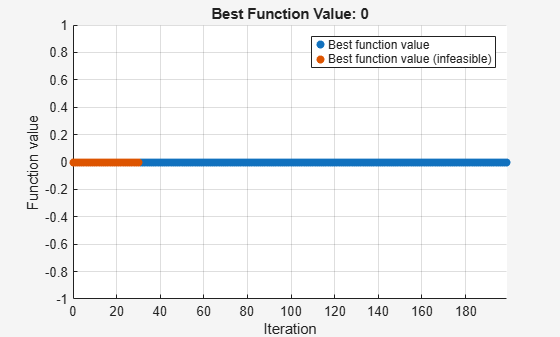# Solve Feasibility Problem Using `surrogateopt`, Problem-Based

Some problems require you to find a point that satisfies all constraints, with no objective function to minimize. For example, suppose that you have the following constraints:

`$\begin{array}{l}\left(y+{x}^{2}{\right)}^{2}+0.1{y}^{2}\le 1\\ y\le \mathrm{exp}\left(-x\right)-3\\ y\le x-4.\end{array}$`

Do any points $\left(x,y\right)$ satisfy the constraints? To answer this question, you need to evaluate the expressions at a variety of points. The `surrogateopt` solver does not require you to provide initial points, and it searches a wide set of points. So, `surrogateopt` works well for feasibility problems.

To visualize the constraints, see Visualize Constraints. For a solver-based approach to this problem, see Solve Feasibility Problem.

Note: This example uses two helper functions, `outfun` and `evaluateExpr`. The code for each function is provided at the end of this example. Make sure the code for each function is included at the end of your script or in a file on the path.

### Set Up Feasibility Problem

For the problem-based approach, create optimization variables `x` and `y`, and create expressions for the listed constraints. To use the `surrogateopt` solver, you must set finite bounds for all variables. Set lower bounds of –10 and upper bounds of 10.

```x = optimvar("x","LowerBound",-10,"UpperBound",10); y = optimvar("y","LowerBound",-10,"UpperBound",10); cons1 = (y + x^2)^2 + 0.1*y^2 <= 1; cons2 = y <= exp(-x) - 3; cons3 = y <= x - 4;```

Create an optimization problem and include the constraints in the problem.

`prob = optimproblem("Constraints",[cons1 cons2 cons3]);`

The problem has no objective function. Internally, the solver sets the objective function value to `0` for every point.

### Solve Problem

Solve the problem using `surrogateopt`.

```rng(1) % For reproducibility [sol,fval] = solve(prob,"Solver","surrogateopt")```
```Solving problem using surrogateopt. ``````surrogateopt stopped because it exceeded the function evaluation limit set by 'options.MaxFunctionEvaluations'. ```
```sol = struct with fields: x: 1.7087 y: -2.8453 ```
```fval = 0 ```

The first several evaluated points are infeasible, as indicated by the color red in the plot. After about 60 evaluations, the solver finds a feasible point, plotted in blue.

Check the feasibility at the returned solution.

`infeasibility(cons1,sol)`
```ans = 0 ```
`infeasibility(cons2,sol)`
```ans = 0 ```
`infeasibility(cons3,sol)`
```ans = 0 ```

All infeasibilities are zero, indicating that the point `sol` is feasible.

### Stop Solver at First Feasible Point

To reach a solution faster, create an output function (see Output Function) that stops the solver whenever it reaches a feasible point. The `outfun` helper function at the end of this example stops the solver when it reaches a point with no constraint violation.

Solve the problem using the `outfun` output function.

```opts = optimoptions("surrogateopt","OutputFcn",@outfun); rng(1) % For reproducibility [sol,fval] = solve(prob,"Solver","surrogateopt","Options",opts)```
```Solving problem using surrogateopt. ``````Optimization stopped by a plot function or output function. ```
```sol = struct with fields: x: 1.7087 y: -2.8453 ```
```fval = 0 ```

This time, the solver stops earlier than before.

### Visualize Constraints

To visualize the constraints, plot the points where each constraint function is zero by using `fimplicit`. The `fimplicit` function passes numeric values to its functions, whereas the `evaluate` function requires a structure. To tie these functions together, use the `evaluateExpr` helper function, which appears at the end of this example. This function simply puts passed values into a structure with the appropriate names.

Avoid a warning that occurs because the `evaluateExpr` function does not work on vectorized inputs. Plot the returned solution point as a green circle.

```s = warning('off','MATLAB:fplot:NotVectorized'); figure cc1 = (y + x^2)^2 + 0.1*y^2 - 1; fimplicit(@(a,b)evaluateExpr(cc1,a,b),[-2 2 -4 2],'r') hold on cc2 = y - exp(-x) + 3; fimplicit(@(a,b)evaluateExpr(cc2,a,b),[-2 2 -4 2],'k') cc3 = y - x + 4; fimplicit(@(x,y)evaluateExpr(cc3,x,y),[-2 2 -4 2],'b') plot(sol.x,sol.y,'og') hold off````warning(s);`

The feasible region is inside the red outline and below the black and blue lines. The feasible region is at the lower right of the red outline.

### Helper Functions

This code creates the `outfun` helper function.

```function stop = outfun(~,optimValues,state) stop = false; switch state case 'iter' if optimValues.currentConstrviolation <= 0 stop = true; end end end```

This code creates the `evaluateExpr` helper function.

```function p = evaluateExpr(expr,x,y) pt.x = x; pt.y = y; p = evaluate(expr,pt); end```